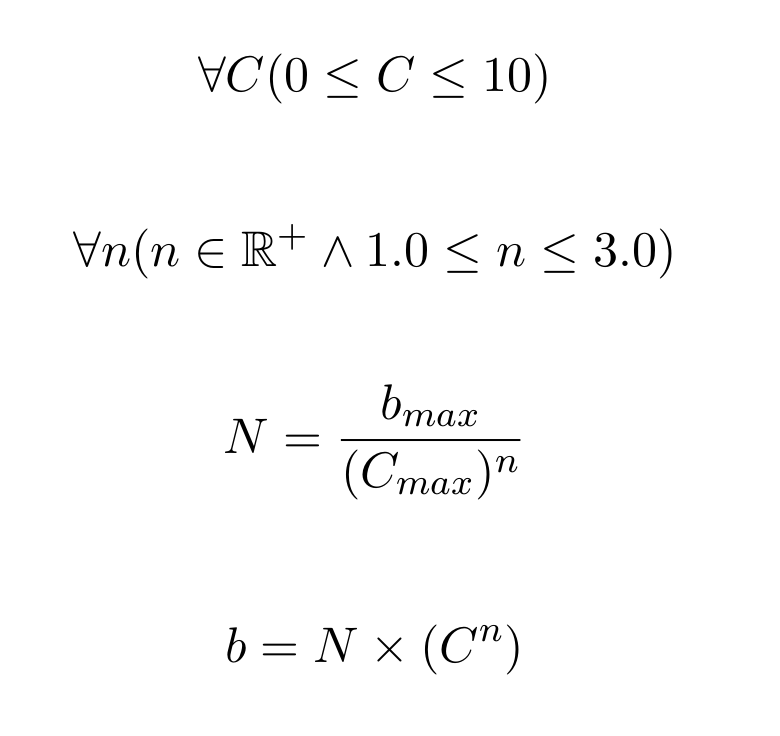This repository has been archived by the owner on Mar 27, 2021. It is now read-only.
/ bounty-formula Public archive

A formula to calculate bounty amounts.

# EdOverflow/bounty-formula

Switch branches/tags
Nothing to show

A tag already exists with the provided branch name. Many Git commands accept both tag and branch names, so creating this branch may cause unexpected behavior. Are you sure you want to create this branch?

## Files

Failed to load latest commit information.
Type
Name
Commit time

# Bounty Formula

A formula to calculate bounty amounts by @TomNomNom and @EdOverflow. The write-up is located here: https://edoverflow.com/2017/the-math-behind-bug-bounties/# MathJax

$$\forall C (0 \le C \le 10)$$

$$\forall n (n \in \Bbb{R}^+ \land 1.0 \le n \le 3.0)$$

$$N = \frac{b_{max}}{(C_{max})^n}$$

$$b = N \times (C^n)$$


# LaTeX

$\forall C(0 \le C \le 10)$

$\forall n (n \in \mathbb{R}^+ \land 1.0 \le n \le 3.0)$

$N = \frac{b_{max}}{(C_{max})^n}$

$b = N \times (C^n)$


# Contributing

We welcome contributions from the public.

### Using the issue tracker 💡

The issue tracker is the preferred channel for bug reports and features requests.

### Issues and labels 🏷

Our bug tracker utilizes several labels to help organize and identify issues.

### Guidelines for bug reports 🐛

Use the GitHub issue search — check if the issue has already been reported.

A formula to calculate bounty amounts.

## Releases

No releases published

## Packages 0

No packages published

•
•